# Solving MultiStep Equations You will Solve multistep equations

• Slides: 17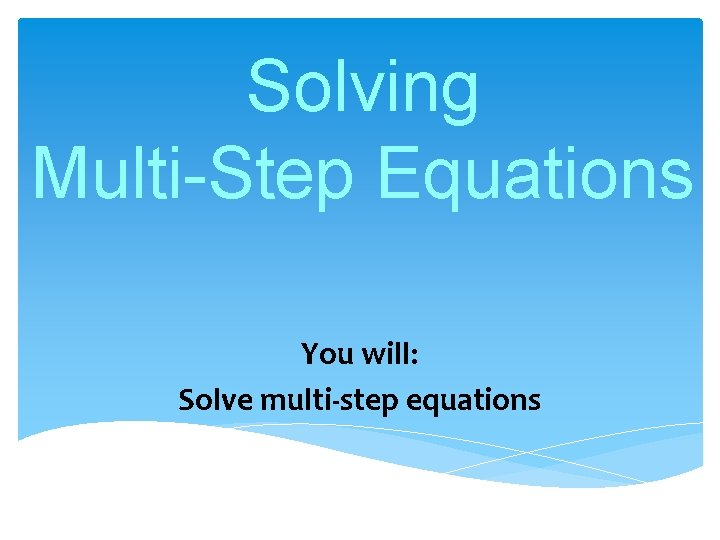Solving Multi-Step Equations You will: Solve multi-step equations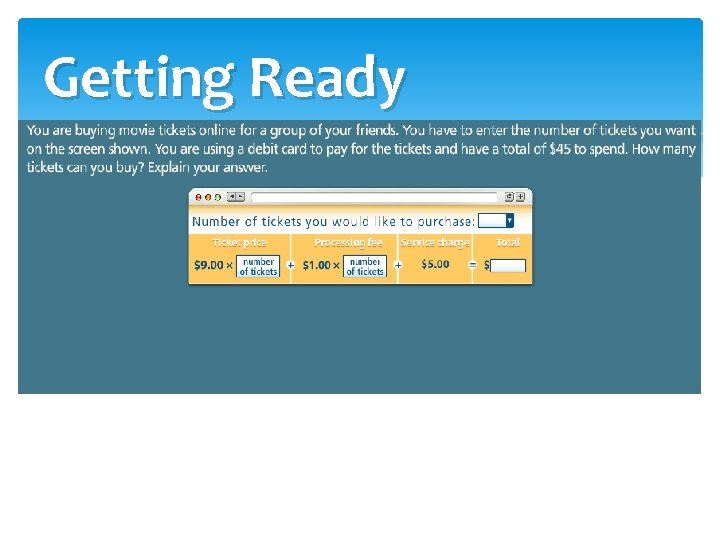Problem 1 a) Solve 5 = 5 m – 23 + 2 m b) Solve -2 y + 5 y= 14 c) Solve 11 m – 8 – 6 m = 22Problem 2 a) Martha takes her niece and nephew to a concert. She buys t-shirts and bumper stickers for them. The bumper stickers cost \$1 each. Martha’s niece wants 1 shirt and 4 bumper stickers, and her nephew wants 2 shirts but no bumper stickers. If Martha’s total is \$67, what is the cost of one shirt?Problem 2 b) Noah and Kate are shopping for new guitar strings in a music store. Noah buys 2 packs of strings. Kate buys 2 packs of strings and a music book. The book costs \$16. Their total cost is \$72. How much is one pack of strings?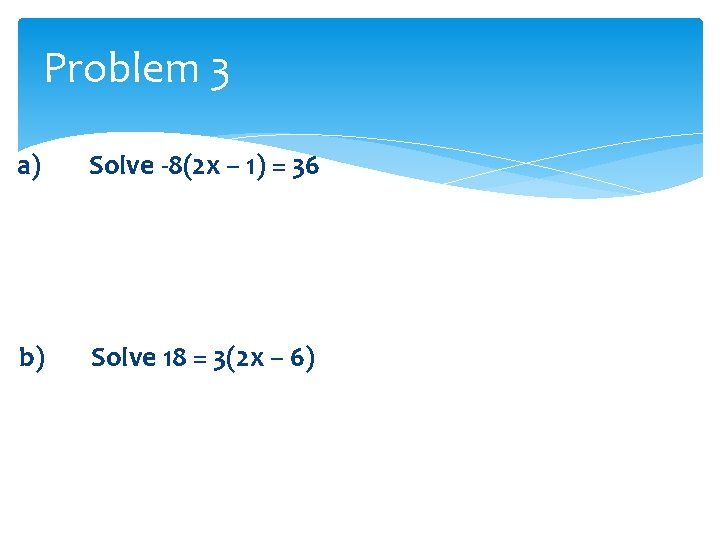Problem 3 a) Solve -8(2 x – 1) = 36 b) Solve 18 = 3(2 x – 6)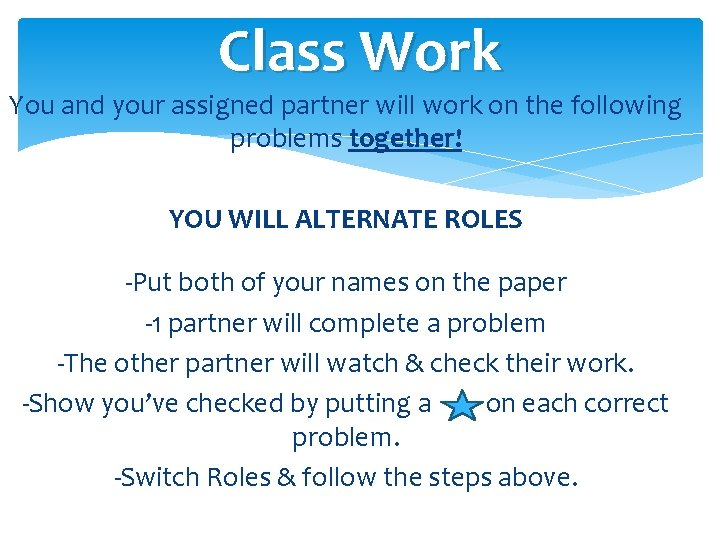Class Work You and your assigned partner will work on the following problems together! YOU WILL ALTERNATE ROLES -Put both of your names on the paper -1 partner will complete a problem -The other partner will watch & check their work. -Show you’ve checked by putting a on each correct problem. -Switch Roles & follow the steps above.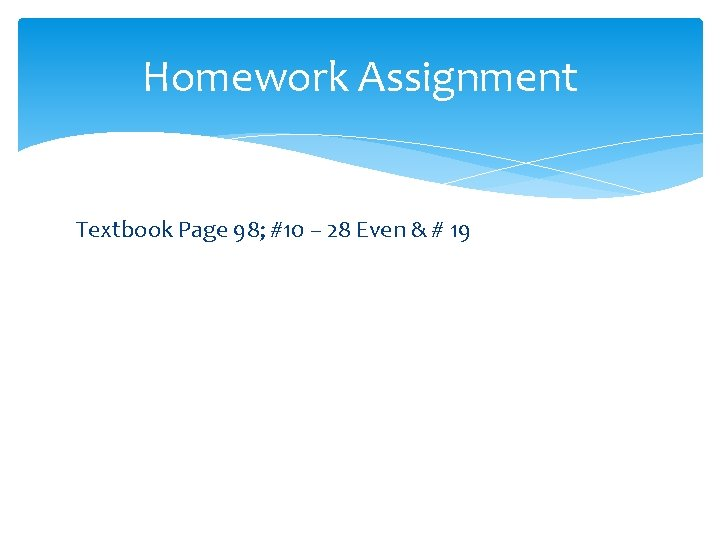Homework Assignment Textbook Page 98; #10 – 28 Even & # 19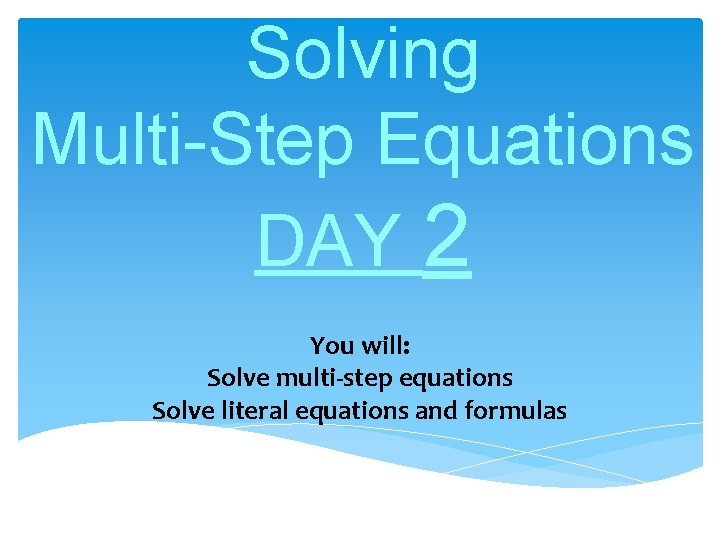Solving Multi-Step Equations DAY 2 You will: Solve multi-step equations Solve literal equations and formulas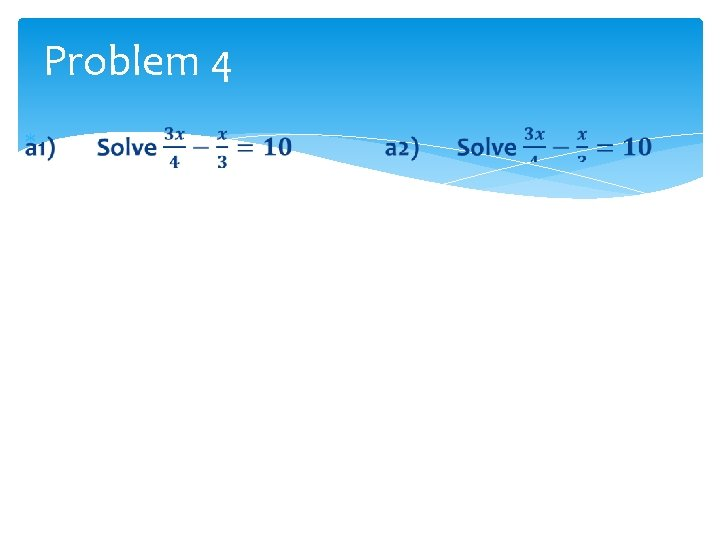Problem 4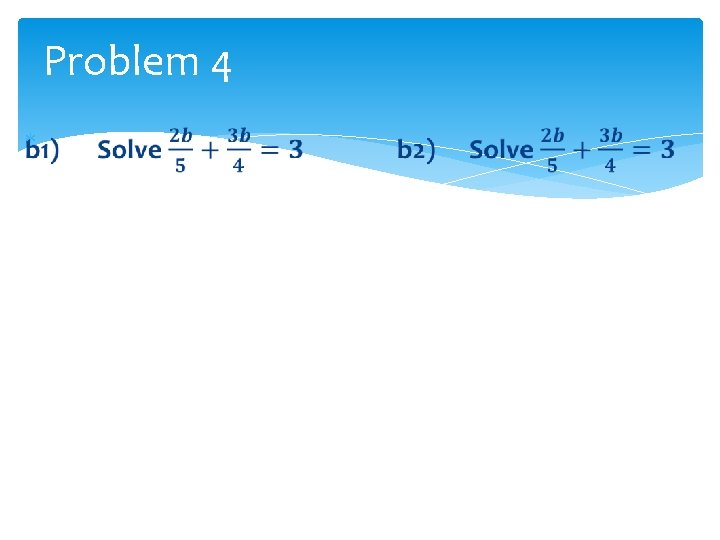Problem 4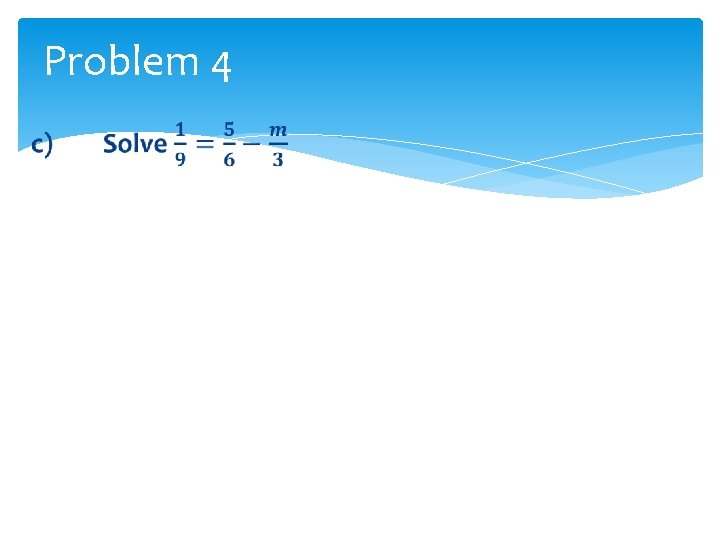Problem 4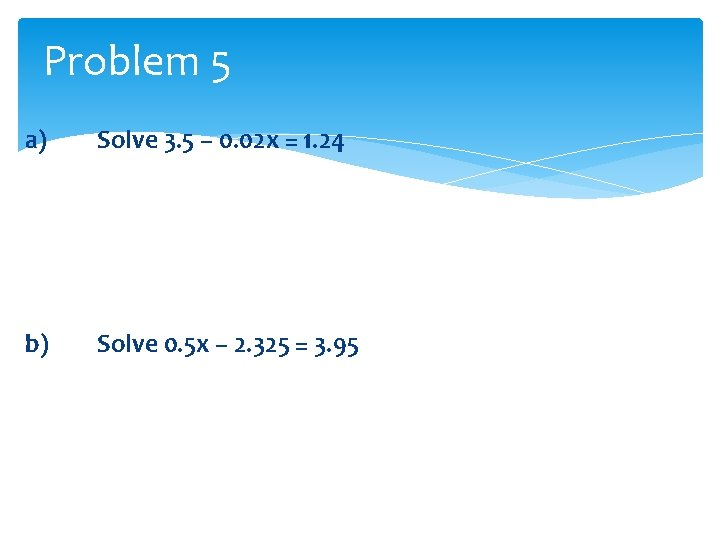Problem 5 a) Solve 3. 5 – 0. 02 x = 1. 24 b) Solve 0. 5 x – 2. 325 = 3. 95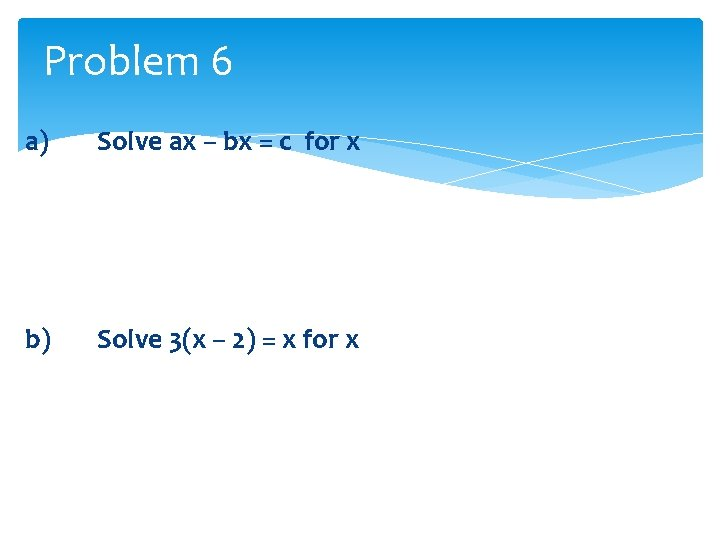Problem 6 a) Solve ax – bx = c for x b) Solve 3(x – 2) = x for x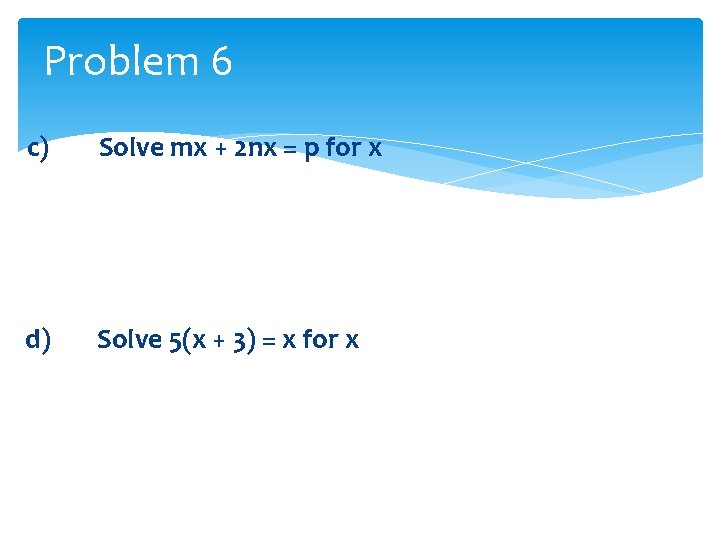Problem 6 c) Solve mx + 2 nx = p for x d) Solve 5(x + 3) = x for x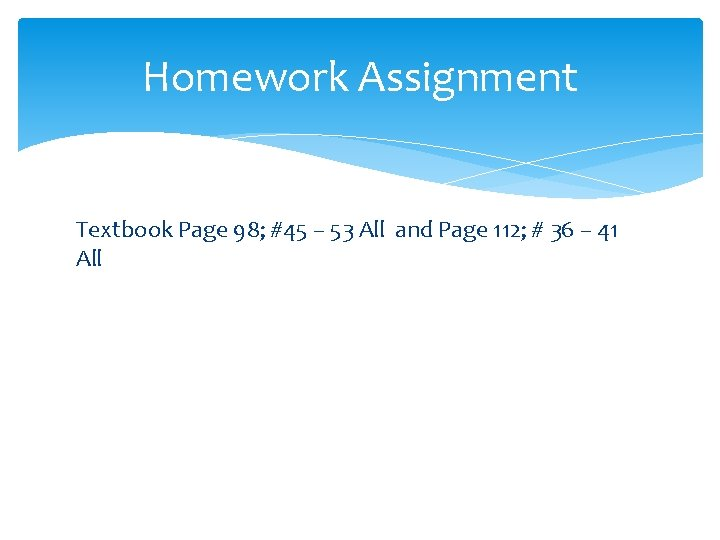Homework Assignment Textbook Page 98; #45 – 53 All and Page 112; # 36 – 41 All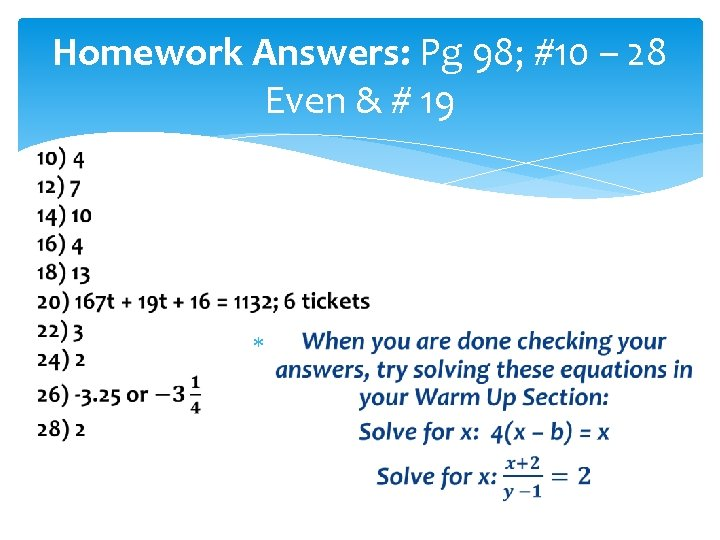Homework Answers: Pg 98; #10 – 28 Even & # 19